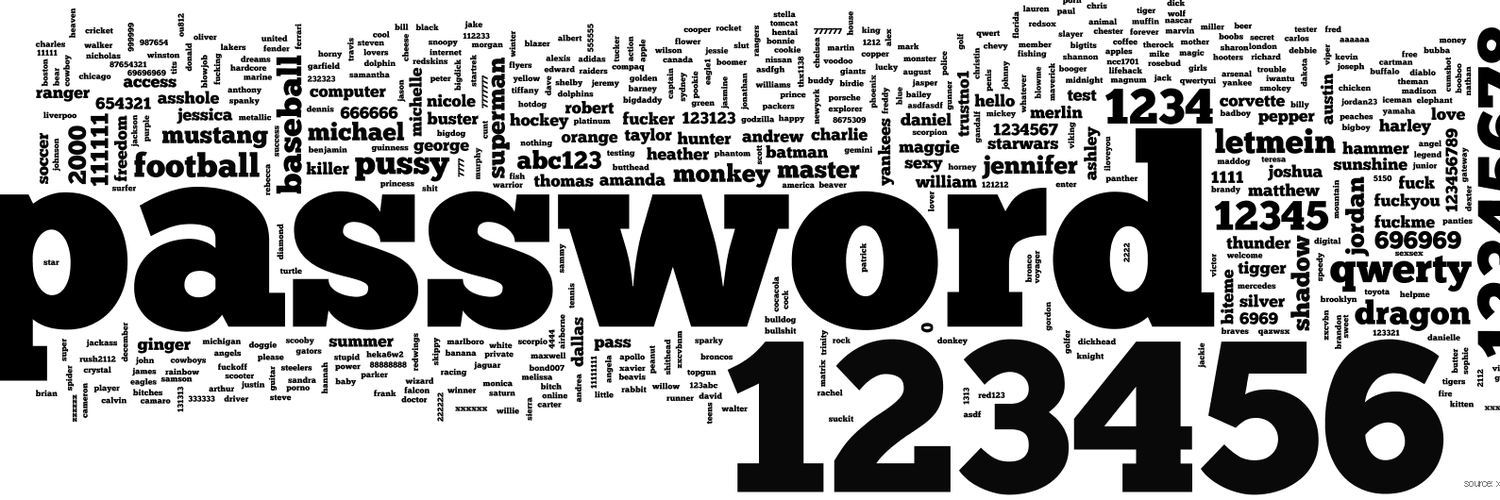0, 1, ... 9, a, b, ... z, A, B, …

00, 01, ... 09, 0 a, ..., 0z, 0A , 0B, …

C = m ^ n

1 95
2 95^2 = 9,025
3 95^3 = 857,375
4 95^4 = 81,450,625
5 95^5 = 7,737,809,375
6 95^6 = 735,091,890,625
7 95^7 = 69,833,729,609,375
8 95^8 = 6,634,204,312,890,625
9 95^9 = 630,249,409,724,609,375
10 95^10 = 59,873,693,923,837,890,625

C = 95 + 95^2 + 95^3 + 95^4 + 95^5 + 95^6 + 95^7 + 95^8 + 95^9 + 95^10 = 60,510,648,114,517,017,120

C = 36^8 = 2,821,109,907,456